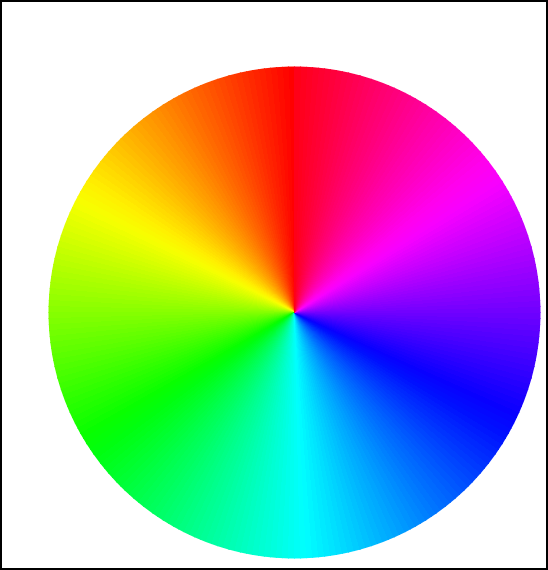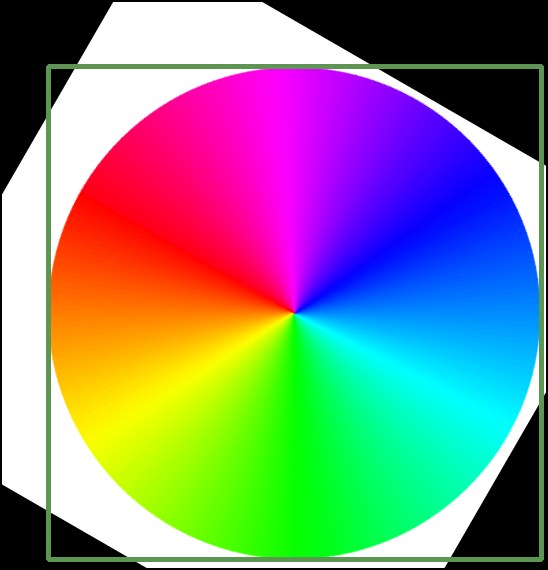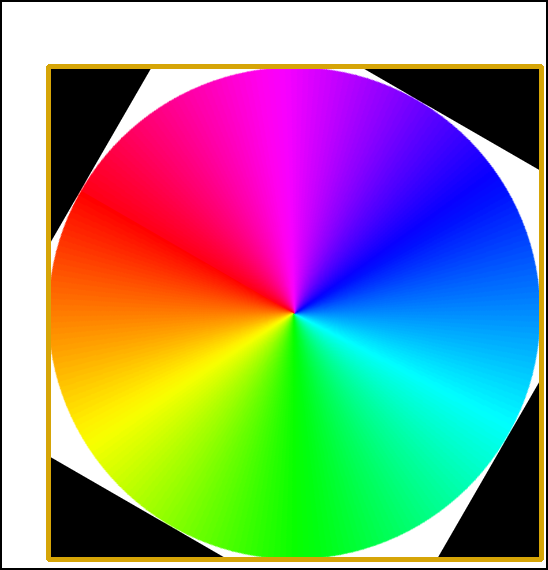/ opencv_wrapper Public

A wrapper library for OpenCV Python

# anbergem/opencv_wrapper

Switch branches/tags
Nothing to show

A tag already exists with the provided branch name. Many Git commands accept both tag and branch names, so creating this branch may cause unexpected behavior. Are you sure you want to create this branch?

## Files

Failed to load latest commit information.
Type
Name
Commit time

# Simple wrapper for opencv-python

OpenCV Wrapper is a simpler wrapper for the `opencv-python` package. As the mentioned package only gives access to OpenCV functions, in a C++ style, it can be tedious to write. There is also no support for the OpenCV classes like Rect, Point etc. OpenCV Wrapper attempts to fix that.

The package is at an early state, and contributions are welcome! The contents of the package have been a demand-and-supply model, where functionality is added as new tedious things in `opencv-python` are found. Do not hesitate to file an issue, requesting new functionality or enhancement proposals!

## Installation

Getting started is easy, using pip or pipenv!

`pip(env) install opencv-wrapper opencv-python`

Note that you must install `opencv-python` separately. The reason is so that you can select the appropriate package from `opencv-python` and `opencv-python-headless`, or instead opt to compile the Python bindings are part of the OpenCV source tree yourself.

## Examples

This code speaks for itself.

Vanilla OpenCV:

```import cv2 as cv
video = cv.VideoCapture("path/to/file")
if not video.isOpened():
raise ValueError("Could not open video")

while True:
if not ok:
break
cv.imshow("Frame", frame)
if cv.waitKey(0) & 0xFF == ord('q'):
break
video.release()```

opencv_wrapper:

```import cv2 as cv
import opencv_wrapper as cvw
for frame in video:
cv.imshow("Frame", frame)
if cvw.wait_key(0) == ord('q'):
break ```

Or, if you want to read a range of frames:

```with cvw.load_video("path/to/file") as video:
for frame in cvw.read_frames(video, start, stop, step):
cv.imshow("Frame", frame)
if cvw.wait_key(0) == ord('q'):
break ```

### Rotate A Color Wheel

Say we have the following color wheel image, which we want to rotate.We of course want to rotate it at it's center, which is not in the center of the image. A possible solution using OpenCV would be

```import cv2 as cv
import random

gray = cv.cvtColor(img, cv.COLOR_BGR2GRAY)
_, otsu = cv.threshold(gray, 250, 255, cv.THRESH_BINARY_INV)
_, contours, _ = cv.findContours(otsu, cv.RETR_EXTERNAL, cv.CHAIN_APPROX_SIMPLE)
contour = contours
rect = cv.boundingRect(contour)  # Gives a tuple (x, y, w, h)
x, y, w, h = rect

color = [random.randint(0, 255) for _ in range(3)]

degrees = 60
center = (x + w / 2), (y + h / 2)
rotation_matrix = cv.getRotationMatrix2D(center, degrees, 1)
rotated_image = cv.warpAffine(img, rotation_matrix, gray.shape[::-1])

cv.rectangle(rotated_image, (x, y), (x + w, y + h), color)

cv.imshow("Image", rotated_image)
cv.waitKey(0)```

We first convert the image to gray scale. The color wheel in gray scale does not contain any values of pure white. We can therefore threshold the image at a high threshold, to segment the color wheel.

We then find contours in the image (which in this case only will be one contour), and find the bounding rectangle enclosing the contour. From this rectangle we can find the center point by the means of the top left corner, the height and width. We use this to create a rotation matrix, and call the affine warp function. Lastly, we draw a rectangle around the found contour. This is just for viewing pruposes.

We get the following result.Although a perfectly fine solution, we cannot help but rotate the whole image. Here is a solution using opencv_wrapper.

opencv_wrapper:

```import cv2 as cv
import opencv_wrapper as cvw

gray = cvw.bgr2gray(img)
otsu = cvw.threshold_binary(gray, 250, inverse=True)
contours = cvw.find_external_contours(otsu)
contour = contours
rect = contour.bounding_rect  # Gives a Rect object
degrees = 60

center = rect.center  # Gives a Point object
top_left = rect.tl  # Gives a Point object
new_center = center - top_left
img[rect.slice] = cvw.rotate_image(
img[rect.slice], new_center, degrees, unit=cvw.AngleUnit.DEGREES
)
cvw.rectangle(img, rect, cvw.Color.RANDOM)

cv.imshow("Image", img)
cvw.wait_key(0)```

We again follow the same approach. However, with the Contour class, we can simply call the bounding rect property. This yields a Rect object, which has a center property. Convenient.

Where we before were left with no (obvious) choice but to rotate the whole image, we can now simply slice the image at the rectangle, only rotating the figure itself. For this exact purpose, it doesn't make much difference, but it is a demonstration. We find the new center from which to rotate, and simply call the rotate image function. We can here choose whether to use degrees or radians. Lastly we draw a rectangle with a random color.

We get the following result.Not only is this a tad less tedious to write, but we are also easily able to rotate only the relevant part of the circle by slicing¹. The contour, rectangle and point objects are also an ease to work with.

### Other Area of Ease

While not providing examples, there are many other parts of the OpenCV that become an ease to work with, when using opencv_wrapper. Areas include

• Morphology
• Image normalization
• Color conversion
• Thresholding
• Image smoothing

¹Disclosure: The slicing is not that hard to accomplish, from `x, y, w, h`. We can create it like this

`our_slice = (slice(y, y+h), slice(x, x+w))`

A wrapper library for OpenCV Python

3 tags

## Packages 0

No packages published

•
•